## Facebook Hacker Cup 2013: round 1 problem analysis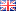Round 1 of the Facebook Hacker Cup has just ended. Competitors were required to solve three nicely-balanced problems: all of them required substantial thought, without being unreasonably difficult. Like last week, I will describe my solutions to the problems, although this time the solution source code is written in C++.

#### Problem 1: Card Game (20 points)

Problem statement here.

The easiest problem in the contest is a combinatorial one. We are given an array A of N distinct integers, and are asked to calculate for each element A[i] how many subsets of A of size K exist, such that A[i] is the largest in the set. Then, we must multiply A[i] by the number of such sets, sum the results, and report the answer modulo 1,000,000,007.

On a high level, this isn't very difficult. If we start by sorting the array A, then we can simply compute the answer as:
• sum A[i] × C(i, K - 1) mod 1,000,000,007 (for 0 ≤ i < N)
... where C(n,r) is the combinatorial choice function that calculates in how many ways we can select r distinct elements from a set of n. The idea behind this formula is that if A is sorted, and A[i] is the largest element, then the other K-1 elements must be drawn from A\..A\[i-1], which are exactly the i elements smaller than A[i].

We can implement this idea in at least three different ways, and the differences are mostly in how we implement the choice function. The most obvious way is to use mathematical definion:
• C(n,k) = n! / k! / (n - k)!
... where x! denotes the factorial of x. This is reasonably efficient if we precalculate the factorials, but it involves very large integers, so we need to use a language that can handle those.

If we want to limit ourselves to 64-bit integer arithmetic only, which is a lot faster, we should perform all calculations modulo 1,000,000,007. But in that case, the division is problematic, because the modulo operator doesn't distribute over division; i.e. generally (x / y) mod z ≠ (x mod z) / (y mod z).

There are two ways to overcome this problem. One is to note that 1,000,000,007 is a prime number, and thus we can calculate the multiplicative inverse of each factorial using e.g. the extended Euclidean algorithm, and multiply with the inverse instead of dividing. This yields on O(N log N) solution, which is pretty good considering that N ≤ 10,000.

During the contest I used a more brute-force approach, however, by simply pre-calculating all relevant binomial coefficients using the recurrence relation:
• C(n,0) = 1
• C(n,n) = 1
• C(n,k) = C(n-1,k-1) + C(n-1,k)
The advantage of this method is that it doesn't require division at all (or multiplication, for that matter). This yields an O(N×N) time and space algorithm, but implemented in C++ this is fast enough, especially considering that the table is independent of the actual input and thus needs to be computed only once.

C++:
 ```1 2 3 4 5 6 7 8 9 10 11 12 13 14 15 16 17 18 19 20 21 22 23 24 25 26 27 28 29 30 31 32 33 34 35 36 37 ``` ```#include #include #include static const int MaxN = 10000, Mod = 1000000007; static int nCr[MaxN+1][MaxN+1]; int main() { // Precalculate binomial coefficients: for (int n = 0; n <= MaxN; ++n) { nCr[n] = nCr[n][n] = 1; for (int r = 1; r < n; ++r) nCr[n][r] = (nCr[n - 1][r] + nCr[n - 1][r - 1])%Mod; } int cases = 0; std::cin >> cases; for (int caseno = 1; caseno <= cases; ++caseno) { // Read input: int N = 0, K = 0; std::cin >> N >> K; std::vector A(N); for (int i = 0; i < N; ++i) std::cin >> A[i]; // Calculate sum: std::sort(A.begin(), A.end()); int answer = 0; for (int i = K - 1; i < N; ++i) answer = (answer + (long long)nCr[i][K - 1]*A[i])%Mod; std::cout << "Case #" << caseno << ": " << answer << std::endl; } }```

I suspect it is also possible to combine the summation with the calculation of the combinations, resulting in a simpler solution that avoids the above complications.

#### Problem 2: Security (35 points)

Problem statement here.

For the second problem, we must determine the lexicographically (read: alphabetically) smallest key string that is consistent with the output produced by the specified transformation, or output "IMPOSSIBLE" if no valid answer exists.

Practically speaking, we need to fill in the blanks in K1 in such a way that it is possible to rearrange its M parts into K2. The key to solving this problem is to realize that if we can determine whether an answer exists at all, we can use that same method to construct the lexicographically least possible answer by filling in the blanks in K1 from left to right, and from 'a' to 'z'.

We can model the problem as a bipartite graph where each vertex corresponds with the parts of K1 and K2, and an edge exists between two parts if the strings match (in the sense that "ab?" matches "?bc" because on every position all characters match). Then a solution exists if and only if the maximum matching in this graph contains exactly M edges.

For example, if K1="ab???c?ab" and K2="a?ccab?a?" and M=3 (so each key consists of three parts of three characters) then the corresponding graph looks like this: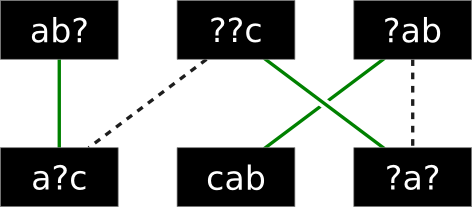There are lines connecting the parts that can be matched together, and the green edges indicate the maximum possible matching. Since all parts are matched, there is a solution for the given pair of keys.

I implemented a maximum matching algorithm based on augmenting paths. How efficient is this approach? We may need to determine if a pair of strings are compatible at most 6×N times (once for each possible blank in K1, multiplied by the number of possible letters: a-f).

For each such case, we must construct a bipartite graph which will have at most M×M edges and find upto M augmenting paths. Finding a single augmenting path requires going through all edges at most once, so this part of the algorithm takes O(M^3) time.

The total runtime of the algorithm is therefore O(6*N*M^3), or about 75 million steps in the worst case, which is acceptable.

C++:
 ```1 2 3 4 5 6 7 8 9 10 11 12 13 14 15 16 17 18 19 20 21 22 23 24 25 26 27 28 29 30 31 32 33 34 35 36 37 38 39 40 41 42 43 44 45 46 47 48 49 50 51 52 53 54 55 56 57 58 59 60 61 62 63 64 65 66 67 68 69 70 71 72 73 74 75 76 77 78 79 80 81 82 83 84 85 86 87 88 89 90 91 92 93 94 95 ``` ```#include #include #include static std::vector > adj; static std::vector matchL, matchR; static std::vector visited; static bool match(int i) { if (visited[i]) return false; visited[i] = true; for (size_t n = 0; n < adj[i].size(); ++n) { const int j = adj[i][n]; if (matchR[j] < 0 || match(matchR[j])) { matchL[i] = j; matchR[j] = i; return true; } } return false; } static bool match_one() { for (int i = 0; i < adj.size(); ++i) { if (matchL[i] < 0 && match(i)) return true; } return false; } static int maximum_matching() { int res = 0; matchL.assign(adj.size(), -1); matchR.assign(adj.size(), -1); while (visited.assign(adj.size(), 0), match_one()) ++res; return res; } static bool compatible(const std::string &s, const std::string &t) { for (size_t i = 0; i < s.size(); ++i) { if (s[i] != '?' && t[i] != '?' && s[i] != t[i]) return false; } return true; } static bool possible(const std::string &K1, const std::string &K2, int M) { int L = K1.size() / M; adj.assign(M, std::vector()); for (int i = 0; i < M; ++i) { for (int j = 0; j < M; ++j) { if (compatible(K1.substr(L*i, L), K2.substr(L*j, L))) adj[i].push_back(j); } } return maximum_matching() == M; } int main() { int cases = 0; std::cin >> cases; for (int caseno = 1; caseno <= cases; ++caseno) { int M; std::string K1, K2; std::cin >> M >> K1 >> K2; std::cout << "Case #" << caseno << ": "; if (!possible(K1, K2, M)) { std::cout << "IMPOSSIBLE" << std::endl;; continue; } for (std::string::iterator it = K1.begin(); it != K1.end(); ++it) { if (*it == '?') { *it = 'a'; while (!possible(K1, K2, M)) ++*it; } } std::cout << K1 << std::endl; } }```

#### Problem 3: Dead Pixels (45 points)

Problem statement here.

For the third problem, I used a brute-force approach again. The constraints are such that it is barely feasible to iterate over all possible window placements, except that we must be able to determine if each placement is possible nearly instantly, or we'll run out of time.

The key to solving this problem is to first reduce it from two dimensions to one. Suppose the height of the window to be placed (Q) is equal to the height of the screen (H). Then, the Y-coordinate of dead pixels doesn't matter, and we are only interested in which columns are blocked by a dead pixel. If we iterate over the blocked columns from left to right, we can easily track the gaps between dead columns; if we find a gap of width G, then we can place a window in the gap if its width P ≤ G. In fact, there are exactly (G - P + 1) ways to place the window in the gap.

To extend this idea to two dimensions, we start by assuming the window is placed against the top of the screen. We can then count how many dead pixels occur in each column for the first Q rows of the screen (with 0-based indices 0 through Q-1), and scan the columns for gaps as described above, counting all valid placements where the top of the window is at Y-coordinate 0. If we move the window down one row (to Y-coordinate 1), then we should update the count of dead pixels per column by adding the dead pixels on row Q and subtracting the dead pixels on row 0 (which no overlap the window). We can repeat this process until we reach the bottom of the screen.

This solution requires Θ(W×H) time. Since W,H ≤ 40,000 that could take a whopping 1,600,000,000 operations per test case; that's a lot! Fortunately, each operation is very simple, so implemented in C++ this solution takes no more than a few seconds per testcase. I wouldn't want to try this in a scripting language, though!

C++:
 ```1 2 3 4 5 6 7 8 9 10 11 12 13 14 15 16 17 18 19 20 21 22 23 24 25 26 27 28 29 30 31 32 33 34 35 36 37 38 39 40 41 42 43 44 45 46 47 48 49 50 51 52 53 54 55 56 57 ``` ```#include #include int main() { int cases = 0; std::cin >> cases; for (int caseno = 1; caseno <= cases; ++caseno) { // Read input (that's a lot of parameters!) int W, H, P, Q, N, X, Y, a, b, c, d; std::cin >> W >> H >> P >> Q >> N >> X >> Y >> a >> b >> c >> d; // Generate a list of dead pixels (grouped by row) std::vector > dead(H); for (int x = X, y = Y, i = 0; i < N; ++i) { int nx = (x*a + y*b + 1)%W, ny = (x*c + y*d + 1)%H; dead[y].push_back(x); x = nx; y = ny; } // Scan row by row, tracking which columns are blocked by dead pixels: std::vector blocked(W + 1); blocked[W] = 1; for (int y = 0; y < Q - 1; ++y) { for (std::vector::iterator it = dead[y].begin(); it != dead[y].end(); ++it) ++blocked[*it]; } int answer = 0; for (int y = 0; y + Q <= H; ++y) { // Add bottom row for (std::vector::iterator it = dead[y + Q - 1].begin(); it != dead[y + Q - 1].end(); ++it) ++blocked[*it]; // Count number of valid horizontal placements: int edge = P; for (int c = 0; c <= W; ++c) { if (blocked[c]) { if (edge <= c) answer += c - edge + 1; edge = c + 1 + P; } } // Remove top row for (std::vector::iterator it = dead[y].begin(); it != dead[y].end(); ++it) --blocked[*it]; } std::cout << "Case #" << caseno << ": " << answer << std::endl; } }```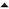02-'13 High-Color GIF Images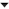01-'13 Facebook Hacker Cup 2013: qualification round problem analysis

How do you come up with these solutions ?

In problem 1 declareer je 'answer' en gebruik je 'res', dat geeft een compiler-error.
Bij opgave 1 en 3 krijg ik dezelfde output, bij 2 zit ik denk ik fout (ik heb o.a. veel meer 'IMPOSSIBLE', ik verwachtte al dat dat niet klopte maar had in de 6 min. geen tijd om uit te zoeken wat er mis ging).

@Eärendil:
You're right, I'll fix that.
Deathraven wrote on Sunday 03 February 2013 @ 21:16:
How do you come up with these solutions ?
To summarize very generally, I like to approach these problems in a top-down fashion, by starting from the abstract description and then breaking it down into slightly simpler subproblems that I may or may not yet know how to solve. Then I try to break those subproblems down until I have something that works.

When that happens, the solution may still have problems: maybe it isn't fast enough, or it uses too much memory, so I try to iteratively improve it until I arrive at a solution that I predict will run in time. The input bounds given in the problem statements are extremely useful to estimate when the solution has become “good enough”.

Of course, it helps if you have some previous exposure to some of these solution techniques. For problem A, it really helps to know at least a little about combinatorics and modular arithmetic. For problem B, you probably have to know what bipartite matching is. By comparison, problem C requires more inventiveness and less background knowledge. In a contest like this you can look stuff up on Wikipedia or Google, but that only works if you know what you're searching for.

Finally, you should realize that the solutions I post here are the result of several rounds of iterative improvement, as described above. The right idea doesn't magically pop into my head, even though it may look like that when you only see the end result and not the process that lead up to it.

I wonder whether the O(W*H) "Pixels" solution was intended to pass or not. It seems far too easy, particularly compared to the graph algorithms needed to solve "Security", but then why set such low constraints when W=H=400,000 would have completely ruled out such solutions while still allowing O(W*logH) sweepline solutions to pass easily?

Perhaps the idea was to rule out O(W*H*N) algorithms, but then wouldn't W=H=1000, N=10^6 have worked for that too?

I believe the solution of Problem 3 in this post is correct.
I have a completely different solution and generated the same result.
https://gist.github.com/4705327

BTW, I think the time complexity code in this post is O(W*H+N).
(N matters when both W and H is small)
The time complexity is O(W + N*log(N))
In my MacBookPro, it's 57.3s v.s. 25.7s.

However, the algorithm in this post is much easier to implement.
For algorithm contest, It's a very big advantage.
It's wise to choose a slower-but-easier-but-passable solution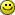Robin Lee: I don't really understand it either. Maybe Facebook will clarify this in their analysis, but I wouldn't be surprised if they just didn't think things through. Fortunately (?) the point values don't really matter in this round considering that a perfect score is (probably) required to advance.

Ok, apparently point values do matter. Looks like 503 people solved Security and 925 people solved Dead Pixels, so that sounds like the Security should have had the highest point value.

miaout17: Thanks for sharing that solution! With regards to the time complexity of my approach: I think you may have missed that the problem statement guarantees N ≤ W×H, so O(W×H + N) = O(W×H).

[Comment edited on maandag 4 februari 2013 08:56]

Cool, thanks for analysis.

I think your solution to question 2 is wrong - there may be several maximum matchings and you have to pick the one which allows for the best possible value for k.

Consider these strings;

k1 = b?
k2 = ?b

We can match the letters either way around, but one gets k = ba, and one gets k = bb

... wat heeft dit met facebook te doen? die link snap ik niet

For problem 1,

you do not have to compute all of C(i,k-1)'s because you can symbolicaly solve coefficent A for which

C(i,k-1) * A = C(i+1,k-)

in terms of i,k and N, which saves many Combination computations.

I wrote a recursive backtracking solution to problem 2 that I think is easier to understand than the bipartite matching. It now runs in 4 seconds on the judged input, but I didn't have it correctly optimized during my 6 minute timer so for that and other reasons I didn't make it to round 2.

The key optimizations were to sort the sections of k2, consider non-wilds (not ?) first, and prune matching sections of k2 and sections of k that won't lead to a better result than the best one already found.

The code that you gave for first problem is giving " java.lang.outofMemoryError".. can u plz suggest how to rectify this error...

hello sir,
you had wrote that "It's wise to choose a slower-but-easier-but-passable solution "
that means that i should not worry about how efficient my algorithm is ...
i just think about solution & i have done???

harsh wrote on Thursday 07 February 2013 @ 03:04:
The code that you gave for first problem is giving " java.lang.outofMemoryError".. can u plz suggest how to rectify this error...
Since the code Soultaker posted in the blog is C++, and you have a Java problem, maybe you can add a link to your own code, then someone might be able to give you some suggestions.
Have you tried allocating more memory to Java (-Xmx1024m)?

[Comment edited on donderdag 7 februari 2013 13:26]

himlims_ wrote on Monday 04 February 2013 @ 13:44:
... wat heeft dit met facebook te doen? die link snap ik niet
ik miste de link ook even. lees meer info hier: